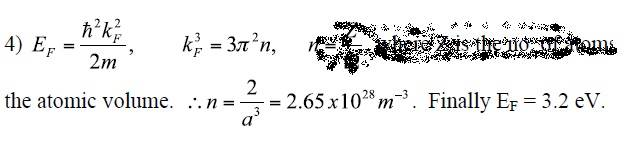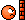# Always calculating Fermi energy incorrectly

## Homework Statement

This isn't really a homework question but I didn't know where to put it.

## Homework Equations

##E_f = \frac{h^2 k_f^2}{2m}##
##k_f = (3 \pi^2n)^{\frac{1}{3}}##

## The Attempt at a Solution

I'm going through a lot of examples and every time I punch the numbers in I get an incorrect result.

For example, I'm looking at the below solution, which has come from a typical 'find the Fermi energy' question. The image shows the final stage of the problem, where n has been found and all that's left to do is plug it into the given Fermi energy equation.However my attempt comes out like so:

##E_f = \frac{\hbar^2 k_f^2}{2m} = \frac{\hbar^2(3\pi^2 2.65*10^28)^{\frac{2}{3}}}{2m} = \frac{(6.63*10^{-34})^2(3\pi^2*2.65*10^{28})^{\frac{2}{3}}}{2*9.1*10^{-31}} = 2.05*10^{-17}##

##\frac{2.05*10^{-17}}{1.6*10^{-19}}= 128eV##

## \neq 3.2eV##

What's going wrong??? I'm making a similar mistake on every Fermi Energy problem I do. The one common error I'm noticing is that my answers always seem to be out be a factor of ##10^{-2}##

Where is the glaring, basic textbook error I'm making here? Something to do with using the correct units? An incorrect value for one of the constants? I think I need a second pair of eyes to look this over for me.

Thanks for any help.

vela
Staff Emeritus
Homework Helper
•Thanks